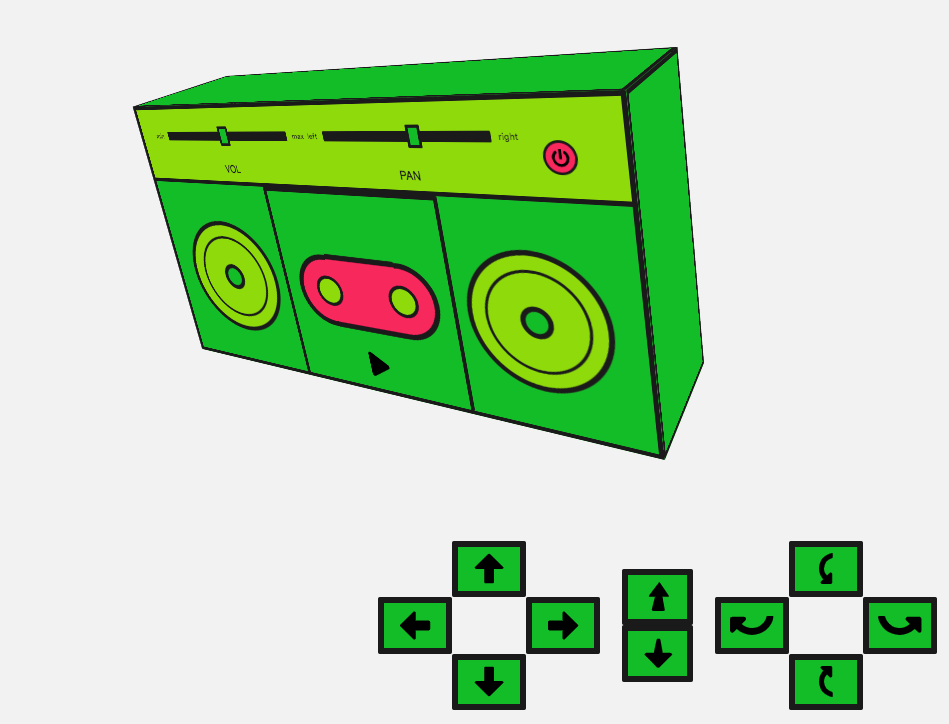# Web audio 空间化基础

## 3D boombox 演示## 创建 audio 收听者

js

``````const AudioContext = window.AudioContext || window.webkitAudioContext;
const audioCtx = new AudioContext();
const listener = audioCtx.listener;

const posX = window.innerWidth / 2;
const posY = window.innerHeight / 2;
const posZ = 300;

listener.positionX.value = posX;
listener.positionY.value = posY;
listener.positionZ.value = posZ - 5;
``````

js

``````listener.forwardX.value = 0;
listener.forwardY.value = 0;
listener.forwardZ.value = -1;
listener.upX.value = 0;
listener.upY.value = 1;
listener.upZ.value = 0;
``````

## 创建 panner 节点

`equalpower` — 计算出默认和一般方式的平移。

`HRTF` — 这代表 'Head-related transfer function' ，在弄清楚声音的位置时，会考虑人脑（对声音的处理）。

js

``````const pannerModel = "HRTF";
``````

js

``````const innerCone = 60;
const outerCone = 90;
const outerGain = 0.3;
``````

js

``````const distanceModel = "linear";
``````

js

``````const maxDistance = 10000;
``````

js

``````const refDistance = 1;
``````

```const rollOff = 10;
```

js

``````const positionX = posX;
const positionY = posY;
const positionZ = posZ;

const orientationX = 0.0;
const orientationY = 0.0;
const orientationZ = -1.0;
``````

js

``````const panner = new PannerNode(audioCtx, {
panningModel: pannerModel,
distanceModel: distanceModel,
positionX: positionX,
positionY: positionY,
positionZ: positionZ,
orientationX: orientationX,
orientationY: orientationY,
orientationZ: orientationZ,
refDistance: refDistance,
maxDistance: maxDistance,
rolloffFactor: rollOff,
coneInnerAngle: innerCone,
coneOuterAngle: outerCone,
coneOuterGain: outerGain,
});
``````

## 移动 boombox

js

``````const moveControls = document
.querySelector("#move-controls")
.querySelectorAll("button");
const boombox = document.querySelector(".boombox-body");

// the values for our css transforms
let transform = {
xAxis: 0,
yAxis: 0,
zAxis: 0.8,
rotateX: 0,
rotateY: 0,
};

// set our bounds
const topBound = -posY;
const bottomBound = posY;
const rightBound = posX;
const leftBound = -posX;
const innerBound = 0.1;
const outerBound = 1.5;
``````

js

``````function moveBoombox(direction) {
switch (direction) {
case "left":
if (transform.xAxis > leftBound) {
transform.xAxis -= 5;
panner.positionX.value -= 0.1;
}
break;
case "up":
if (transform.yAxis > topBound) {
transform.yAxis -= 5;
panner.positionY.value -= 0.3;
}
break;
case "right":
if (transform.xAxis < rightBound) {
transform.xAxis += 5;
panner.positionX.value += 0.1;
}
break;
case "down":
if (transform.yAxis < bottomBound) {
transform.yAxis += 5;
panner.positionY.value += 0.3;
}
break;
}
}
``````

js

``````case 'back':
if (transform.zAxis > innerBound) {
transform.zAxis -= 0.01;
panner.positionZ.value += 40;
}
break;
case 'forward':
if (transform.zAxis < outerBound) {
transform.zAxis += 0.01;
panner.positionZ.value -= 40;
}
break;
``````

js

``````// set up rotation constants
const rotationRate = 60; // bigger number equals slower sound rotation

const q = Math.PI / rotationRate; //rotation increment in radians
``````

js

``````// get degrees for css
const degreesX = (q * 180) / Math.PI;
const degreesY = (q * 180) / Math.PI;
``````

js

``````case 'rotate-left':
transform.rotateY -= degreesY;

// 'left' is rotation about y-axis with negative angle increment
z = panner.orientationZ.value*Math.cos(q) - panner.orientationX.value*Math.sin(q);
x = panner.orientationZ.value*Math.sin(q) + panner.orientationX.value*Math.cos(q);
y = panner.orientationY.value;

panner.orientationX.value = x;
panner.orientationY.value = y;
panner.orientationZ.value = z;
break;
``````

js

``````case 'rotate-right':
transform.rotateY += degreesY;
// 'right' is rotation about y-axis with positive angle increment
z = panner.orientationZ.value*Math.cos(-q) - panner.orientationX.value*Math.sin(-q);
x = panner.orientationZ.value*Math.sin(-q) + panner.orientationX.value*Math.cos(-q);
y = panner.orientationY.value;
panner.orientationX.value = x;
panner.orientationY.value = y;
panner.orientationZ.value = z;
break;
case 'rotate-up':
transform.rotateX += degreesX;
// 'up' is rotation about x-axis with negative angle increment
z = panner.orientationZ.value*Math.cos(-q) - panner.orientationY.value*Math.sin(-q);
y = panner.orientationZ.value*Math.sin(-q) + panner.orientationY.value*Math.cos(-q);
x = panner.orientationX.value;
panner.orientationX.value = x;
panner.orientationY.value = y;
panner.orientationZ.value = z;
break;
case 'rotate-down':
transform.rotateX -= degreesX;
// 'down' is rotation about x-axis with positive angle increment
z = panner.orientationZ.value*Math.cos(q) - panner.orientationY.value*Math.sin(q);
y = panner.orientationZ.value*Math.sin(q) + panner.orientationY.value*Math.cos(q);
x = panner.orientationX.value;
panner.orientationX.value = x;
panner.orientationY.value = y;
panner.orientationZ.value = z;
break;
``````

js

``````function moveBoombox(direction, prevMove) {
switch (direction) {
case "left":
if (transform.xAxis > leftBound) {
transform.xAxis -= 5;
panner.positionX.value -= 0.1;
}
break;
case "up":
if (transform.yAxis > topBound) {
transform.yAxis -= 5;
panner.positionY.value -= 0.3;
}
break;
case "right":
if (transform.xAxis < rightBound) {
transform.xAxis += 5;
panner.positionX.value += 0.1;
}
break;
case "down":
if (transform.yAxis < bottomBound) {
transform.yAxis += 5;
panner.positionY.value += 0.3;
}
break;
case "back":
if (transform.zAxis > innerBound) {
transform.zAxis -= 0.01;
panner.positionZ.value += 40;
}
break;
case "forward":
if (transform.zAxis < outerBound) {
transform.zAxis += 0.01;
panner.positionZ.value -= 40;
}
break;
case "rotate-left":
transform.rotateY -= degreesY;

// 'left' is rotation about y-axis with negative angle increment
z =
panner.orientationZ.value * Math.cos(q) -
panner.orientationX.value * Math.sin(q);
x =
panner.orientationZ.value * Math.sin(q) +
panner.orientationX.value * Math.cos(q);
y = panner.orientationY.value;

panner.orientationX.value = x;
panner.orientationY.value = y;
panner.orientationZ.value = z;
break;
case "rotate-right":
transform.rotateY += degreesY;
// 'right' is rotation about y-axis with positive angle increment
z =
panner.orientationZ.value * Math.cos(-q) -
panner.orientationX.value * Math.sin(-q);
x =
panner.orientationZ.value * Math.sin(-q) +
panner.orientationX.value * Math.cos(-q);
y = panner.orientationY.value;
panner.orientationX.value = x;
panner.orientationY.value = y;
panner.orientationZ.value = z;
break;
case "rotate-up":
transform.rotateX += degreesX;
// 'up' is rotation about x-axis with negative angle increment
z =
panner.orientationZ.value * Math.cos(-q) -
panner.orientationY.value * Math.sin(-q);
y =
panner.orientationZ.value * Math.sin(-q) +
panner.orientationY.value * Math.cos(-q);
x = panner.orientationX.value;
panner.orientationX.value = x;
panner.orientationY.value = y;
panner.orientationZ.value = z;
break;
case "rotate-down":
transform.rotateX -= degreesX;
// 'down' is rotation about x-axis with positive angle increment
z =
panner.orientationZ.value * Math.cos(q) -
panner.orientationY.value * Math.sin(q);
y =
panner.orientationZ.value * Math.sin(q) +
panner.orientationY.value * Math.cos(q);
x = panner.orientationX.value;
panner.orientationX.value = x;
panner.orientationY.value = y;
panner.orientationZ.value = z;
break;
}

boombox.style.transform =
"translateX(" +
transform.xAxis +
"px) translateY(" +
transform.yAxis +
"px) scale(" +
transform.zAxis +
") rotateY(" +
transform.rotateY +
"deg) rotateX(" +
transform.rotateX +
"deg)";

const move = prevMove || {};
move.frameId = requestAnimationFrame(() => moveBoombox(direction, move));
return move;
}
``````

## 连接我们的控件

js

``````// for each of our controls, move the boombox and change the position values
moveControls.forEach(function (el) {
let moving;
"mousedown",
function () {
let direction = this.dataset.control;
if (moving && moving.frameId) {
window.cancelAnimationFrame(moving.frameId);
}
moving = moveBoombox(direction);
},
false,
);

"mouseup",
function () {
if (moving && moving.frameId) {
window.cancelAnimationFrame(moving.frameId);
}
},
false,
);
});
``````